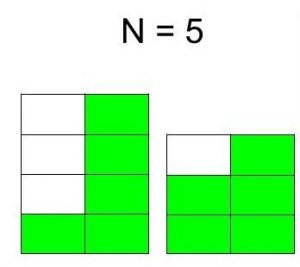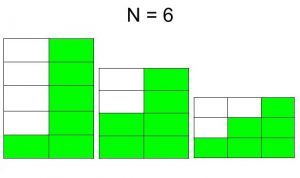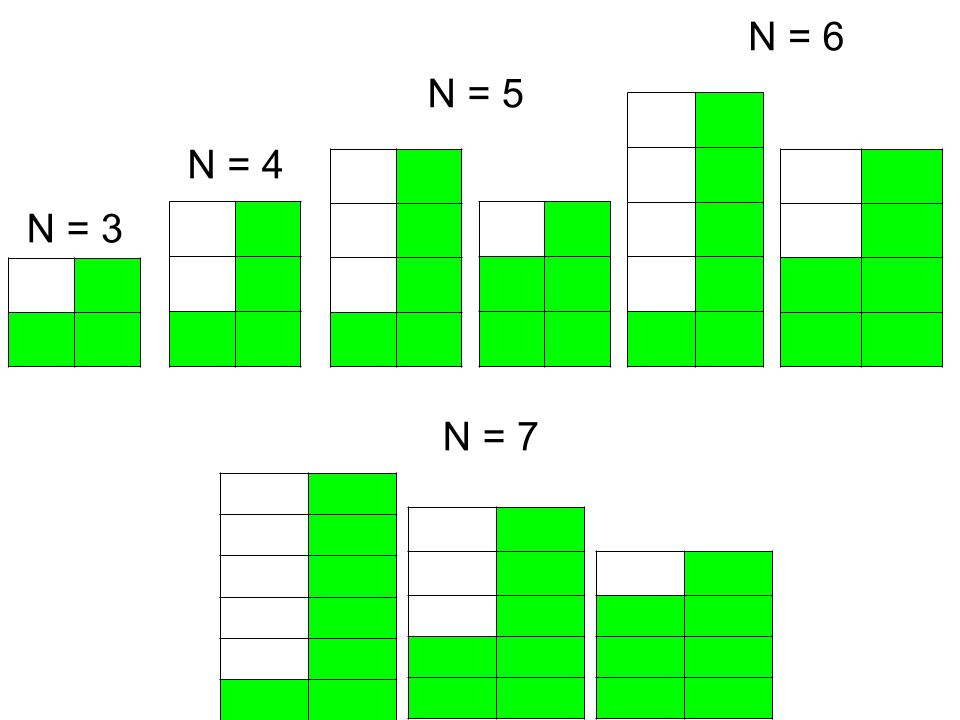# Total number of different staircase that can made from N boxes

Given N boxes each of unit dimension i.e. (1×1). The task is to find the total number of different staircases that can be made from those boxes with the following rules:

• Staircase must be in strictly descending order.
• Each staircase contains at least two steps. (Total steps is equal to the breadth of the staircase.)

Examples:

Input : N = 5
Output : 2
The two staircase are following :Input : N = 6
Output : 3
The three staircase are following :## Recommended: Please try your approach on {IDE} first, before moving on to the solution.

If we consider total steps = 2, we can observe the fact that the number of staircase is incremented by 1 if N is incremented by 2. We can illustrate the above thing from the following image :Now, if total steps is greater than 2 (assume, total steps = K) then we can take this thing as first create a base (base requires boxes equal to the total steps) for staircase and put another staircase on it of steps size K and K – 1 having boxes N – K. (because K boxes already used to create base). Thus, we can solve this problem using bottom-up dynamic programming.

Below is the implementation of the above approach:

## C++

 `// C++ program to find the total number of ` `// different staircase that can made ` `// from N boxes ` `#include ` `using` `namespace` `std; ` ` `  `// Function to find the total number of ` `// different staircase that can made ` `// from N boxes ` `int` `countStaircases(``int` `N) ` `{ ` `    ``// DP table, there are two states. ` `    ``// First describes the number of boxes ` `    ``// and second describes the step ` `    ``int` `memo[N + 5][N + 5]; ` ` `  `    ``// Initilize all the elements of ` `    ``// the table to zero ` `    ``for` `(``int` `i = 0; i <= N; i++) { ` `        ``for` `(``int` `j = 0; j <= N; j++) { ` `            ``memo[i][j] = 0; ` `        ``} ` `    ``} ` ` `  `    ``// Base case ` `    ``memo = memo = 1; ` ` `  `    ``for` `(``int` `i = 5; i <= N; i++) { ` `        ``for` `(``int` `j = 2; j <= i; j++) { ` ` `  `            ``// When step is equal to 2 ` `            ``if` `(j == 2) { ` `                ``memo[i][j] = memo[i - j][j] + 1; ` `            ``} ` ` `  `            ``// When step is greater than 2 ` `            ``else` `{ ` `                ``memo[i][j] = memo[i - j][j] +  ` `                             ``memo[i - j][j - 1]; ` `            ``} ` `        ``} ` `    ``} ` ` `  `    ``// Count the total staircase ` `    ``// from all the steps ` `    ``int` `answer = 0; ` `    ``for` `(``int` `i = 1; i <= N; i++)  ` `        ``answer = answer + memo[N][i];     ` ` `  `    ``return` `answer; ` `} ` ` `  `// Driver Code ` `int` `main() ` `{ ` `    ``int` `N = 7; ` ` `  `    ``cout << countStaircases(N); ` ` `  `    ``return` `0; ` `} `

## Java

 `// Java program to find the total number of ` `// different staircase that can made ` `// from N boxes ` ` `  `import` `java.util.*; ` ` `  `class` `GFG ` `{ ` `        ``// Function to find the total number of ` `        ``// different staircase that can made ` `        ``// from N boxes ` `        ``static` `int` `countStaircases(``int` `N) ` `        ``{ ` `            ``// DP table, there are two states. ` `            ``// First describes the number of boxes ` `            ``// and second describes the step ` `            ``int` `[][] memo=``new` `int``[N + ``5``][N + ``5``]; ` `         `  `            ``// Initilize all the elements of ` `            ``// the table to zero ` `            ``for` `(``int` `i = ``0``; i <= N; i++) { ` `                ``for` `(``int` `j = ``0``; j <= N; j++) { ` `                    ``memo[i][j] = ``0``; ` `                ``} ` `            ``} ` `         `  `            ``// Base case ` `            ``memo[``3``][``2``] = memo[``4``][``2``] = ``1``; ` `         `  `            ``for` `(``int` `i = ``5``; i <= N; i++) { ` `                ``for` `(``int` `j = ``2``; j <= i; j++) { ` `         `  `                    ``// When step is equal to 2 ` `                    ``if` `(j == ``2``) { ` `                        ``memo[i][j] = memo[i - j][j] + ``1``; ` `                    ``} ` `         `  `                    ``// When step is greater than 2 ` `                    ``else` `{ ` `                        ``memo[i][j] = memo[i - j][j] +  ` `                                    ``memo[i - j][j - ``1``]; ` `                    ``} ` `                ``} ` `            ``} ` `         `  `            ``// Count the total staircase ` `            ``// from all the steps ` `            ``int` `answer = ``0``; ` `            ``for` `(``int` `i = ``1``; i <= N; i++)  ` `                ``answer = answer + memo[N][i];  ` `         `  `            ``return` `answer; ` `        ``} ` `         `  `        ``// Driver Code ` `        ``public` `static` `void` `main(String [] args) ` `        ``{ ` `            ``int` `N = ``7``; ` `         `  `            ``System.out.println(countStaircases(N)); ` `         `  `             `  `        ``} ` ` `  `} ` ` `  `// This code is contributed  ` `// by ihritik `

## Python 3

 `# Python 3 program to find the total  ` `# number of different staircase that  ` `# can made from N boxes ` ` `  `# Function to find the total number  ` `# of different staircase that can  ` `# made from N boxes ` `def` `countStaircases(N): ` ` `  `    ``# DP table, there are two states. ` `    ``# First describes the number of boxes ` `    ``# and second describes the step ` `    ``memo ``=` `[[``0` `for` `x ``in` `range``(N ``+` `5``)] ` `               ``for` `y ``in` `range``(N ``+` `5``)] ` ` `  `    ``# Initilize all the elements of ` `    ``# the table to zero ` `    ``for` `i ``in` `range``(N ``+` `1``): ` `        ``for` `j ``in` `range` `(N ``+` `1``): ` `            ``memo[i][j] ``=` `0` `         `  `    ``# Base case ` `    ``memo[``3``][``2``] ``=` `memo[``4``][``2``] ``=` `1` ` `  `    ``for` `i ``in` `range` `(``5``, N ``+` `1``) : ` `        ``for` `j ``in` `range` `(``2``, i ``+` `1``) : ` ` `  `            ``# When step is equal to 2 ` `            ``if` `(j ``=``=` `2``) : ` `                ``memo[i][j] ``=` `memo[i ``-` `j][j] ``+` `1` `             `  `            ``# When step is greater than 2 ` `            ``else` `: ` `                ``memo[i][j] ``=` `(memo[i ``-` `j][j] ``+`  `                              ``memo[i ``-` `j][j ``-` `1``]) ` `     `  `    ``# Count the total staircase ` `    ``# from all the steps ` `    ``answer ``=` `0` `    ``for` `i ``in` `range` `(``1``, N ``+` `1``): ` `        ``answer ``=` `answer ``+` `memo[N][i]  ` ` `  `    ``return` `answer ` ` `  `# Driver Code ` `if` `__name__ ``=``=` `"__main__"``: ` ` `  `    ``N ``=` `7` ` `  `    ``print` `(countStaircases(N)) ` ` `  `# This code is contributed ` `# by ChitraNayal `

## C#

 `// C# program to find the total number  ` `// of different staircase that can made ` `// from N boxes ` `using` `System; ` ` `  `class` `GFG ` `{ ` `     `  `// Function to find the total number  ` `// of different staircase that can  ` `// made from N boxes ` `static` `int` `countStaircases(``int` `N) ` `{ ` `    ``// DP table, there are two states. ` `    ``// First describes the number of boxes ` `    ``// and second describes the step ` `    ``int` `[,] memo = ``new` `int``[N + 5, N + 5]; ` ` `  `    ``// Initilize all the elements  ` `    ``// of the table to zero ` `    ``for` `(``int` `i = 0; i <= N; i++)  ` `    ``{ ` `        ``for` `(``int` `j = 0; j <= N; j++) ` `        ``{ ` `            ``memo[i, j] = 0; ` `        ``} ` `    ``} ` ` `  `    ``// Base case ` `    ``memo[3, 2] = memo[4, 2] = 1; ` ` `  `    ``for` `(``int` `i = 5; i <= N; i++) ` `    ``{ ` `        ``for` `(``int` `j = 2; j <= i; j++)  ` `        ``{ ` ` `  `            ``// When step is equal to 2 ` `            ``if` `(j == 2)  ` `            ``{ ` `                ``memo[i, j] = memo[i - j, j] + 1; ` `            ``} ` ` `  `            ``// When step is greater than 2 ` `            ``else`  `            ``{ ` `                ``memo[i, j] = memo[i - j, j] +  ` `                             ``memo[i - j, j - 1]; ` `            ``} ` `        ``} ` `    ``} ` ` `  `    ``// Count the total staircase ` `    ``// from all the steps ` `    ``int` `answer = 0; ` `    ``for` `(``int` `i = 1; i <= N; i++)  ` `        ``answer = answer + memo[N, i];  ` ` `  `    ``return` `answer; ` `} ` ` `  `// Driver Code ` `public` `static` `void` `Main() ` `{ ` `    ``int` `N = 7; ` ` `  `    ``Console.WriteLine(countStaircases(N)); ` `} ` `} ` ` `  `// This code is contributed  ` `// by Subhadeep `

## PHP

 ` `

Output:

```4
```

Time Complexity: O().

Attention reader! Don’t stop learning now. Get hold of all the important DSA concepts with the DSA Self Paced Course at a student-friendly price and become industry ready.

My Personal Notes arrow_drop_upMy name is Saurav Chandra I am from Rajasthan I am currently pursuing my B Tech from National Institute of Technology Hamirpur (H P) in Computer Science and Engineering Department My area of interests are Algorithms and Data Structures

If you like GeeksforGeeks and would like to contribute, you can also write an article using contribute.geeksforgeeks.org or mail your article to contribute@geeksforgeeks.org. See your article appearing on the GeeksforGeeks main page and help other Geeks.

Please Improve this article if you find anything incorrect by clicking on the "Improve Article" button below.